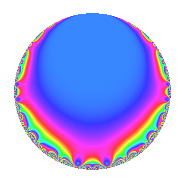# Properties

 Label 90.2.a.aLevel 90 Weight 2 Character orbit 90.a Self dual Yes Analytic conductor 0.719 Analytic rank 0 Dimension 1 CM No Inner twists 1

# Related objects

## Newspace parameters

 Level: $$N$$ = $$90 = 2 \cdot 3^{2} \cdot 5$$ Weight: $$k$$ = $$2$$ Character orbit: $$[\chi]$$ = 90.a (trivial)

## Newform invariants

 Self dual: Yes Analytic conductor: $$0.718653618192$$ Analytic rank: $$0$$ Dimension: $$1$$ Coefficient field: $$\mathbb{Q}$$ Coefficient ring: $$\mathbb{Z}$$ Coefficient ring index: $$1$$ Fricke sign: $$-1$$ Sato-Tate group: $\mathrm{SU}(2)$

## $q$-expansion

 $$f(q)$$ $$=$$ $$q$$ $$\mathstrut -\mathstrut q^{2}$$ $$\mathstrut +\mathstrut q^{4}$$ $$\mathstrut +\mathstrut q^{5}$$ $$\mathstrut +\mathstrut 2q^{7}$$ $$\mathstrut -\mathstrut q^{8}$$ $$\mathstrut +\mathstrut O(q^{10})$$ $$q$$ $$\mathstrut -\mathstrut q^{2}$$ $$\mathstrut +\mathstrut q^{4}$$ $$\mathstrut +\mathstrut q^{5}$$ $$\mathstrut +\mathstrut 2q^{7}$$ $$\mathstrut -\mathstrut q^{8}$$ $$\mathstrut -\mathstrut q^{10}$$ $$\mathstrut +\mathstrut 6q^{11}$$ $$\mathstrut -\mathstrut 4q^{13}$$ $$\mathstrut -\mathstrut 2q^{14}$$ $$\mathstrut +\mathstrut q^{16}$$ $$\mathstrut -\mathstrut 6q^{17}$$ $$\mathstrut -\mathstrut 4q^{19}$$ $$\mathstrut +\mathstrut q^{20}$$ $$\mathstrut -\mathstrut 6q^{22}$$ $$\mathstrut +\mathstrut q^{25}$$ $$\mathstrut +\mathstrut 4q^{26}$$ $$\mathstrut +\mathstrut 2q^{28}$$ $$\mathstrut -\mathstrut 6q^{29}$$ $$\mathstrut -\mathstrut 4q^{31}$$ $$\mathstrut -\mathstrut q^{32}$$ $$\mathstrut +\mathstrut 6q^{34}$$ $$\mathstrut +\mathstrut 2q^{35}$$ $$\mathstrut +\mathstrut 8q^{37}$$ $$\mathstrut +\mathstrut 4q^{38}$$ $$\mathstrut -\mathstrut q^{40}$$ $$\mathstrut +\mathstrut 8q^{43}$$ $$\mathstrut +\mathstrut 6q^{44}$$ $$\mathstrut -\mathstrut 3q^{49}$$ $$\mathstrut -\mathstrut q^{50}$$ $$\mathstrut -\mathstrut 4q^{52}$$ $$\mathstrut -\mathstrut 6q^{53}$$ $$\mathstrut +\mathstrut 6q^{55}$$ $$\mathstrut -\mathstrut 2q^{56}$$ $$\mathstrut +\mathstrut 6q^{58}$$ $$\mathstrut +\mathstrut 6q^{59}$$ $$\mathstrut +\mathstrut 2q^{61}$$ $$\mathstrut +\mathstrut 4q^{62}$$ $$\mathstrut +\mathstrut q^{64}$$ $$\mathstrut -\mathstrut 4q^{65}$$ $$\mathstrut -\mathstrut 4q^{67}$$ $$\mathstrut -\mathstrut 6q^{68}$$ $$\mathstrut -\mathstrut 2q^{70}$$ $$\mathstrut -\mathstrut 12q^{71}$$ $$\mathstrut -\mathstrut 10q^{73}$$ $$\mathstrut -\mathstrut 8q^{74}$$ $$\mathstrut -\mathstrut 4q^{76}$$ $$\mathstrut +\mathstrut 12q^{77}$$ $$\mathstrut -\mathstrut 4q^{79}$$ $$\mathstrut +\mathstrut q^{80}$$ $$\mathstrut +\mathstrut 12q^{83}$$ $$\mathstrut -\mathstrut 6q^{85}$$ $$\mathstrut -\mathstrut 8q^{86}$$ $$\mathstrut -\mathstrut 6q^{88}$$ $$\mathstrut +\mathstrut 12q^{89}$$ $$\mathstrut -\mathstrut 8q^{91}$$ $$\mathstrut -\mathstrut 4q^{95}$$ $$\mathstrut +\mathstrut 2q^{97}$$ $$\mathstrut +\mathstrut 3q^{98}$$ $$\mathstrut +\mathstrut O(q^{100})$$

## Embeddings

For each embedding $$\iota_m$$ of the coefficient field, the values $$\iota_m(a_n)$$ are shown below.

For more information on an embedded modular form you can click on its label.

Label $$\iota_m(\nu)$$ $$a_{2}$$ $$a_{3}$$ $$a_{4}$$ $$a_{5}$$ $$a_{6}$$ $$a_{7}$$ $$a_{8}$$ $$a_{9}$$ $$a_{10}$$
1.1
 0
−1.00000 0 1.00000 1.00000 0 2.00000 −1.00000 0 −1.00000
 $$n$$: e.g. 2-40 or 990-1000 Significant digits: Format: Complex embeddings Normalized embeddings Satake parameters Satake angles

## Inner twists

This newform does not admit any (nontrivial) inner twists.

## Atkin-Lehner signs

$$p$$ Sign
$$2$$ $$1$$
$$3$$ $$1$$
$$5$$ $$-1$$

## Hecke kernels

This newform can be constructed as the intersection of the kernels of the following linear operators acting on $$S_{2}^{\mathrm{new}}(\Gamma_0(90))$$:

 $$T_{7}$$ $$\mathstrut -\mathstrut 2$$ $$T_{11}$$ $$\mathstrut -\mathstrut 6$$